# 7+ Metric Conversion Chart Templates For Kids

Kids learn the importance and usage of Metric Conversion Chart Templates from the time they step onto the primary level of education. It is imperative for kids to learn the basic metric conversion chart as mathematical calculations become easier and friendlier this way. Often teachers device small poems and anecdotes to make it easy for the kids to memorize the metric conversion chart. The following charts are simple documents which can be used to teach metric conversion to young children.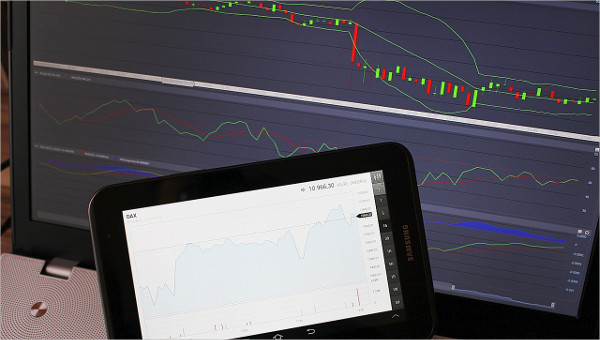## Simple Metric Conversion Chart For Kid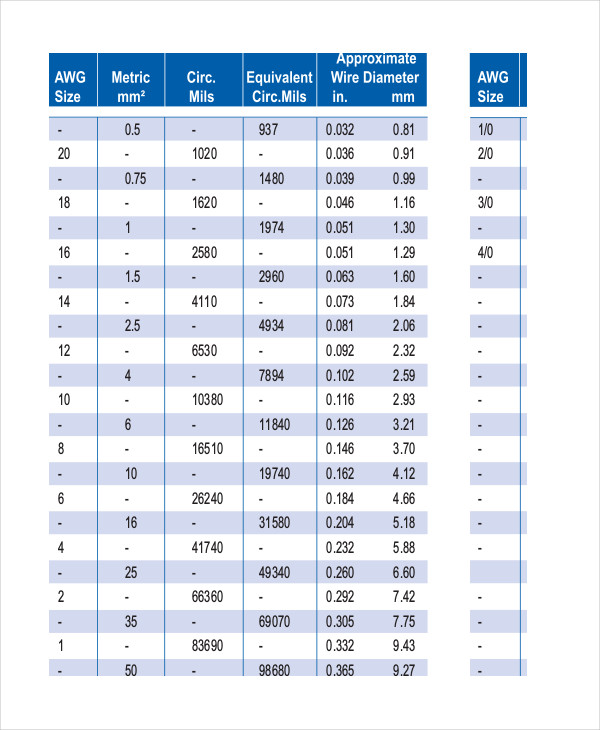andersonpower.com

This metric conversion Chart Templates only comprises of a single unit of metric conversions such as from milligram to gram to kilogram or from millimeter to centimeter, meter, kilometer and likewise.

## Basic Metric Conversion Chart For Kid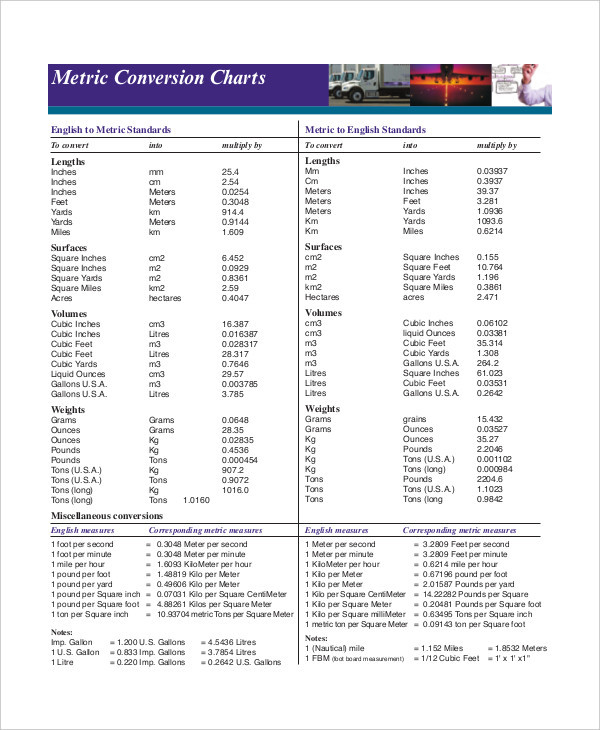stevensglobal.com

The basic metric conversion chart has been devised for kids for them to understand the various modes of conversion of fundamental metric conversion and to be able to perform simple conversion math.

## Kid Conversion Chart For Metric System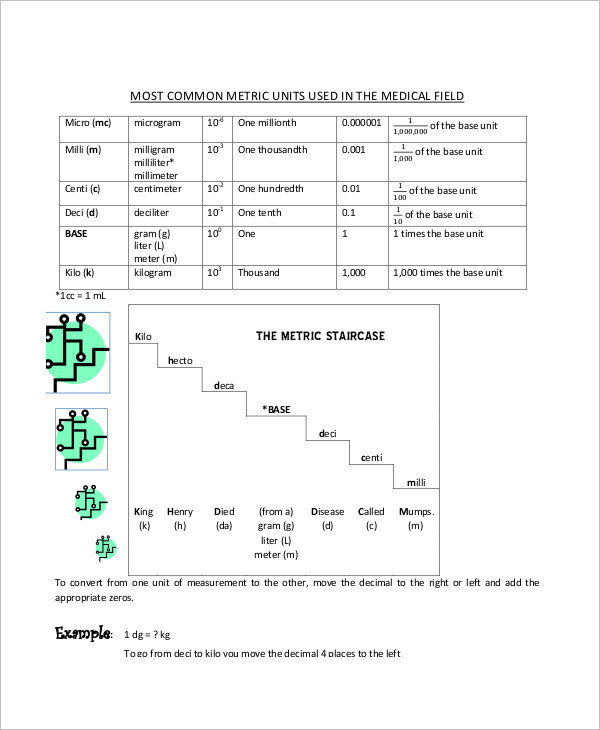laniertech.edu

The Conversion Chart for kids is a printable document you can use to teach young boys and girls the techniques of conversion of basic units of measurement for the metric system.

## Standard To Metric Conversion Chart For Kid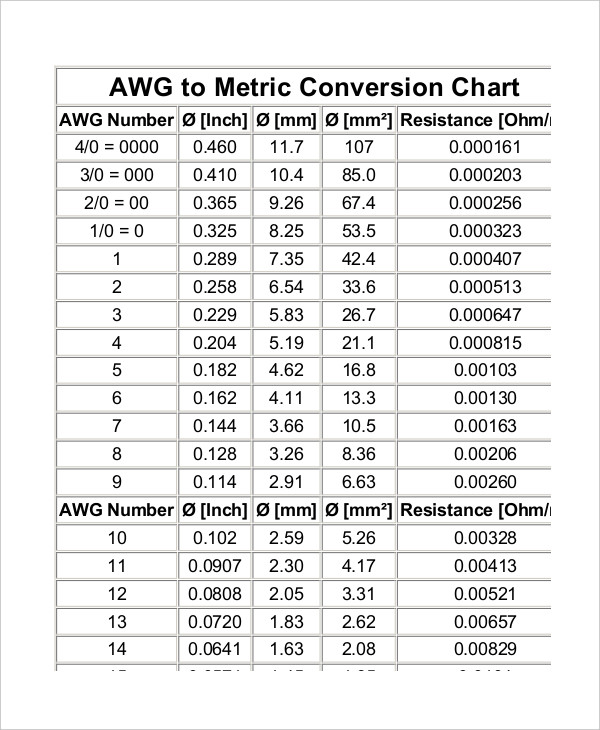victronenergy.com

The Standard to Metric Conversion Chart is used to teach primary level students how to convert different metric units of measure, standard units of measure and conversions between metric and standard.

## Metric Conversion Data Chart for Kid Template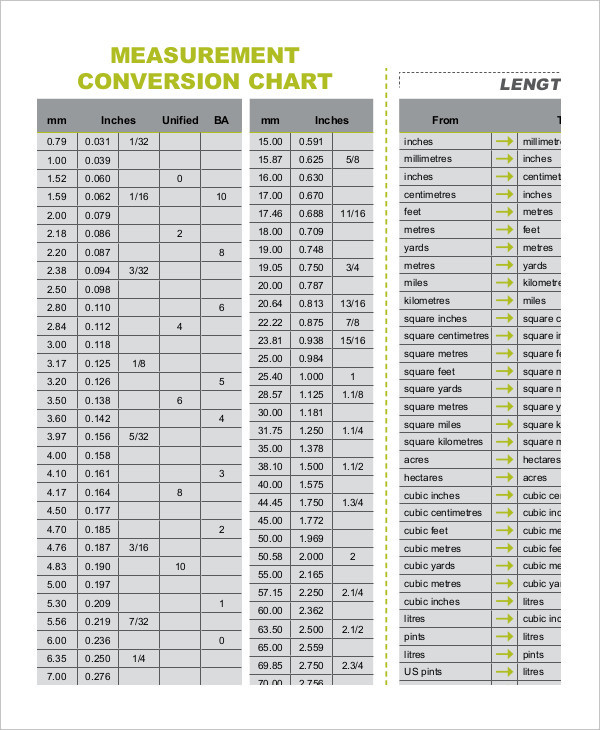meredithdist.com.au

## Metric Measurement Conversion Chart for Kid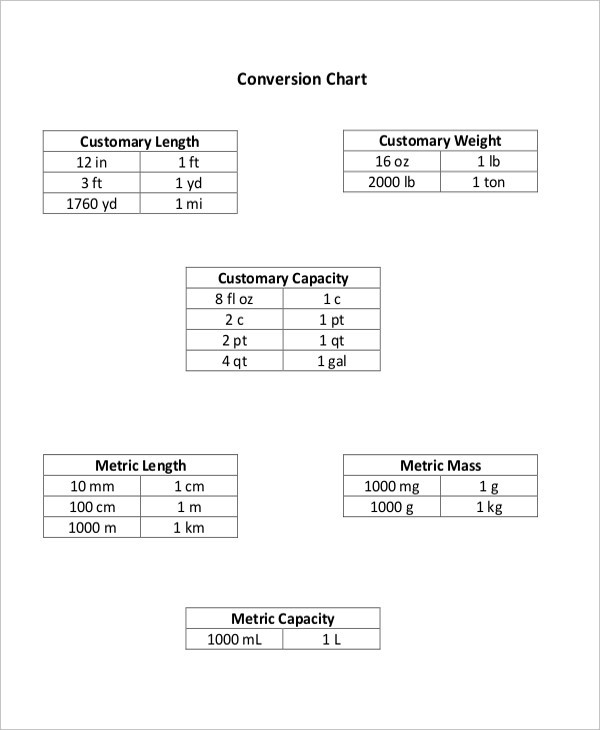wccusd.net

## Kid Metric Length Conversion Chart Template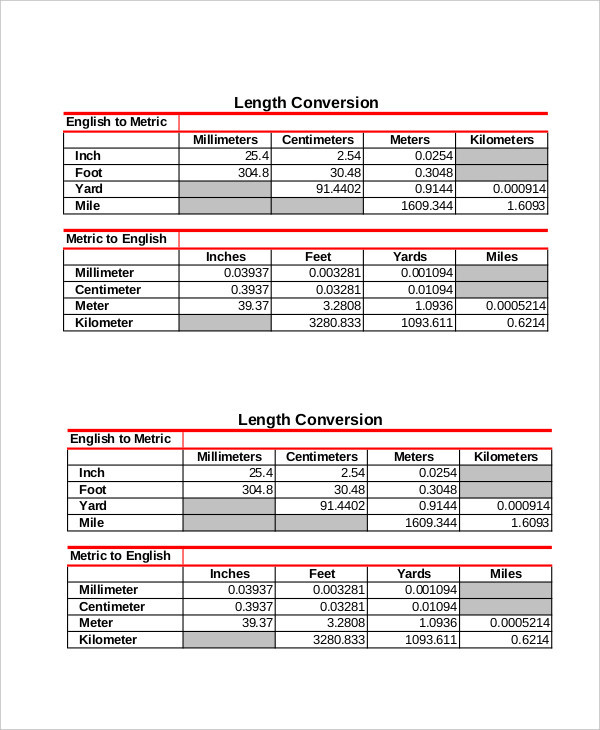labtesting.com

## Metric Conversion Calculator For Kid

This chart can be useful to assist students in understanding and calculating the various modes of units for metric conversion. It also teaches them how to go about making the calculations.

### > How to use the Metric Conversion Chart for kids?

The Metric Conversion Chart for kids is predominantly used by teachers and other instructors to facilitate the knowledge of the Metric system of conversion to students in their classes. Students always remember what they see and therefore, these charts can either be made by hand or printed out to be hanged on the class walls for them to repeatedly look and remember. You can also see Metric System Conversion Chart Templates.

The Metric Conversion Chart for kids comprises of simple units of measurements and refrains from including units such as pressure, speed, light or power. The conversions are usually three of four folds and do not involve complex calculations.

### > What are the advantages of the Metric Conversion chart for kids?

The Metric conversion chart for kids provides for an easy and unique way to make young students and kids memorize the conversion chart, convert and calculate units of measurement. More than often, the chart comprises of simple units of measurements and conversions for units such as length, volume and distance.

The charts can be customized and created for various levels and apart from these personalized charts, you can also add a few worksheets for them to practice conversions. The fractions are expressed in decimal notations which make it easy for the students to understand the math simply by shifting decimal points to the left or right. You can also see Simple Metric Conversion Chart Templates.

Kids learn faster when they see. Therefore, the Metric Conversion Chart for kids is a useful document which the teachers can use to edit and download in order to hang this on the classroom walls so that they can refer to it always and learn the basic unit conversions by heart.